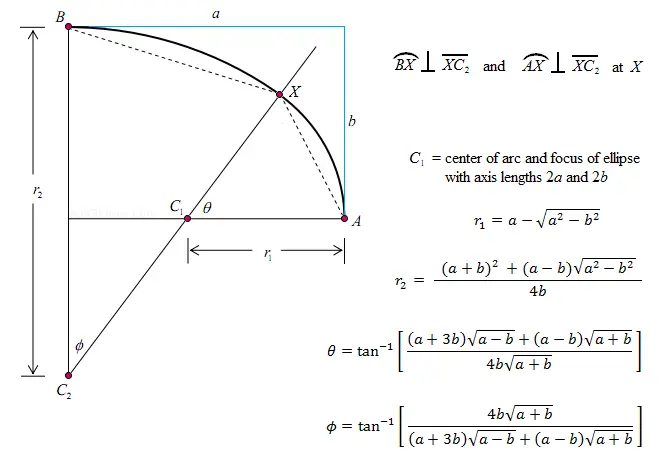# Ellipse Approximation: Method 2

Ellipse Approximation Calculator
half-major axis: a =
half-minor axis: b =
r2 =       r1 =
φ =        θ =

### Finding two circular arcs such that the center of the smaller arc corresponds to the focus of a true ellipse with the same dimensions as the pseudo-ellipse

An ellipse has constantly varying curvature, which is why for some real-world applications it is easier to create curves that approximate true ellipses. The most common way of doing so is with pairs of circular arcs joined at their point of tangency. There is more than one way to choose the radii of the arcs to form a pseudo-ellipse with given dimensions.

For example, you can choose the radii such that the arcs are quarter circles. Or, as explained in this article, you can choose the radii such that the center of the smaller arc coincides with the focus of an ellipse with the same major and minor axis lengths. See diagram below for more detail.Here, a and b are the half-lengths of the major and minor axes respectively. The lengths r₂ and r₁ are the radii of the larger and smaller circular arcs respectively. The points C₂ and C₁ are the respective centers of these circles. X is the point where the two circular arcs meet tangently, and the points X, C₁, and C₂ are collinear.

C₁ is chosen so that it coincides with the focus of an ellipse whose major and minor axis lengths are 2a and 2b.

If c is the distance between the center of the ellipse and one of the foci, then a = √b² + c². This key piece of information allows you to find r₂ and r₁. Once you know r₂ and r₁, you can find their corresponding angles φ and θ. The equations are shown in the image above.

Example: Suppose you want to make a pseudo-ellipse that is 34 inches long and 30 inches wide. Thus, a = 17 and b = 15. Using the formula above, you can compute

r₁ = 17 - sqrt(289-225)
= 17 - sqrt(64)
= 9

r₂ = [(17+15)² + 2*sqrt(289-225)]/[4*15]
= /60
= 17.3333

The angles of these arcs are

φ = arctan{[4*15*sqrt(32)]/[62*sqrt(2) + 2*sqrt(32)]}
= arctan{[4*15*4*sqrt(2)]/[62*sqrt(2) + 2*4*sqrt(2)]}
= arctan{[240*sqrt(2)]/[70*sqrt(2)]}